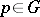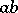# Prime element

(diff) ← Older revision | Latest revision (diff) | Newer revision → (diff)
A generalization of the notion of a prime number. Letbe an integral domain or commutative semi-group with an identity. A non-zero elementthat is not a divisor of unity is called prime if a productcan be divided byonly if one of the elementsorcan be divided by. Every prime element is irreducible, i.e. is divisible only by divisors of unity or elements associated to it. An irreducible element need not be prime; however, in a Gauss semi-group both concepts coincide. Moreover, if every irreducible element of a semi-groupis prime, thenis a Gauss semi-group. Analogous statements hold for a factorial ring. An element of a ring is prime if and only if the principal ideal generated by this element is a prime ideal.